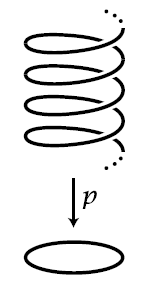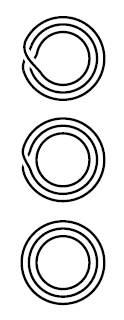Contents

Statement

A basic statement in homotopy theory:

Proposition

(fundamental group of the circle is the integers)

The fundamental group $\pi_1$ of the circle $S^1$ is the additive group of integers:

$\pi_1(S^1) \overset{\simeq}{\longrightarrow} \mathbb{Z}$

and the isomorphism is given by assigning winding number.

Here in the context of topological homotopy theory the circle $S^1$ is the topological subspace $S^1 = \{x \in \mathbb{R}^2 \,\vert\, x_1^2 + x_2^2 = 1 \} \subset \mathbb{R}^2$ of the Euclidean plane with its metric topology, or any topological space of the same homotopy type. More generally, the circle in question is, as a homotopy type, the homotopy pushout

$S^1 \simeq \ast \underset{\ast \sqcup \ast}{\coprod} \ast \,,$

hence the homotopy type with the universal property that it makes a homotopy commuting diagram of the form

$\array{ \ast \sqcup \ast &\longrightarrow& \ast \\ \downarrow &\swArrow& \downarrow \\ \ast &\longrightarrow& S^1 } \,.$

Proofs

In topological point-set homotopy theory

We discuss the classical proof in point-set topological homotopy theory.

ProofThe universal covering space $\widehat{S^1}$ of $S^1$ is the real line (by this example):

$p \coloneqq (cos(2 \pi(-)), \sin(2 \pi(-))) \;\colon\; \mathbb{R}^1 \longrightarrow S^1 \,.$

Since the circle is locally path-connected (this example) and semi-locally simply connected (this example) the fundamental theorem of covering spaces applies and gives that the automorphism group of $\mathbb{R}^1$ over $S^1$ equals the automorphism group of its monodromy permutation representation:

$Aut_{Cov(S^1)}(\mathbb{R}^1) \;\simeq\; Aut_{\pi_1(S^1) Set}(Fib_{S^1}) \,.$

Moreover, as a corollary of the fundamental theorem of covering spaces we have that the monodromy representation of a universal covering space is given by the action of the fundamental group $\pi_1(S)$ on itself (this prop.).

But the automorphism group of any group regarded as an action on itself by left multiplication is canonically isomorphic to that group itself (by this example), hence we have

$Aut_{{\pi_1(S^1)} Set}(Fib_{S^1}) \;\simeq\; Aut_{{\pi_1(S^1)} Set}( \pi_1(S^1) ) \;\simeq\; \pi_1(S^1) \,.$

Therefore to conclude the proof it is now sufficient to show that

$\mathrm{Aut}_{Cov(S^1)}(\mathbb{R}^1) \simeq \mathbb{Z} \,.$

# To that end, consider a homeomorphism of the form

$\array{ \mathbb{R}^1 && \underoverset{\simeq}{f}{\longrightarrow} && \mathbb{R}^1 \\ & {}_{\mathllap{p}}\searrow && \swarrow_{\mathrlap{p}} \\ && S^1 } \,.$

Let $s \in S^1$ be any point, and consider the restriction of $f$ to the fibers over the complement:

$\array{ p^{-1}(S^1 \setminus \{s\}) && \underoverset{\simeq}{f}{\longrightarrow} && p^{-1}(S^1 \setminus \{s\}) \\ & {}_{\mathllap{p}}\searrow && \swarrow_{\mathrlap{p}} \\ && S^1 \setminus \{s\} } \,.$

By the covering space property we have (via this example) a homeomorphism

$p^{-1}(S^1 \setminus \{s\}) \simeq (0,1) \times Disc(\mathbb{Z}) \,.$

Therefore, up to homeomorphism, the restricted function is of the form

$\array{ (0,1)\times Disc(\mathbb{Z}) && \underoverset{\simeq}{f}{\longrightarrow} && (0,1) \times Disc(\mathbb{Z}) \\ & {}_{pr_1}\searrow && \swarrow_{pr_1} \\ && (0,1) } \,.$

By the universal property of the product topological space this means that $f$ is equivalently given by its two components

$(0,1) \times Disc(\mathbb{Z}) \overset{pr_1 \circ f}{\longrightarrow} (0,1) \phantom{AAAA} (0,1) \times Disc(\mathbb{Z}) \overset{pr_2 \circ f}{\longrightarrow} Disc(\mathbb{Z}) \,.$

By the commutativity of the above diagram, the first component is fixed to be $pr_1$. Moreover, by the fact that $Disc(\mathbb{Z})$ is a discrete space it follows that the second component is a locally constant function (by this example). Therefore, since the product space with a discrete space is a disjoint union space (via this example)

$(0,1) \times Disc(\mathbb{Z}) \simeq \underset{n \in \mathbb{Z}}{\sqcup}(0,1)$

and since the disjoint summands $(0,1)$ are connected topological spaces (this example), it follows that the second component is a constant function on each of these summands (by this example).

Finally, since every function out of a discrete topological space is continuous, it follows in conclusion that the restriction of $f$ to the fibers over $S^1 \setminus \{s\}$ is entirely encoded in an endofunction of the set of integers

$\phi \;\colon\; \mathbb{Z} \to \mathbb{Z}$

by

$\array{ S^1 \setminus \{s\} \times Disc(\mathbb{Z}) &\overset{f}{\longrightarrow}& S^1 \setminus \{s\} \times Disc(\mathbb{Z}) \\ (t,k) &\mapsto& (t, \phi(k)) } \,.$

Now let $s' \in S^1$ be another point, distinct from $s$. The same analysis as above applies now to the restriction of $f$ to $S^1 \setminus \{s'\}$ and yields a function

$\phi' \;\colon\; \mathbb{Z} \longrightarrow \mathbb{Z} \,.$

Since

$\left\{ p^{-1}(S^1 \setminus \{s\}) \subset \mathbb{R}^1 \,,\, p^{-1}(S^1 \setminus \{s'\}) \subset \mathbb{R}^1 \right\}$

is an open cover of $\mathbb{R}^1$, it follows that $f$ is unqiuely fixed by its restrictions to these two subsets.

Now unwinding the definition of $p$ shows that the condition that the two restrictions coincide on the intersection $S^1 \setminus \{s,s'\}$ implies that there is $n \in \mathbb{Z}$ such that $\phi(k) = k+ n$ and $\phi'(k) = k+n$.

This shows that $Aut_{Cov(S^1)}(\mathbb{R}^1) \simeq \mathbb{Z}$.

In homotopy type theory

There is also a purely synthetic a proof in homotopy type theory (Licata-Shulman 13, UF, corollary 8.1.10).

Consequences

Example

(isomorphism classes of coverings of the circle are conjugacy classes in the symmetric group)

The monodromy construction assigns to an isomorphism class of covering spaces over the circle $S^1$ with fibers consisting of $n$ elements conjugacy classes of elements the symmetric group $\Sigma(n)$:

$\left \{ \array{ \text{isomorphism classes of} \\ \text{finite covering spaces } \\ \text{over the circle} } \right\} \;\simeq\; \left\{ \array{ \text{conjugacy classes of} \\ \text{elements of a symmetric group} } \right\}$

To see this, we may without restriction (via this prop.) choose a basepoint $x \in S^1$ so that a monodromy representation is equivalently a groupoid morphism of the form

$\rho \;\colon\; B \mathbb{Z} \overset{\simeq}{\longrightarrow} B \pi_1(S^1,x) \overset{\rho}{\longrightarrow} Core(Set) \,.$

Since $\mathbb{Z}$ is the free abelian group on a single generator, such as morphism is uniquely determined by the image of $1 \in \mathbb{Z}$. This is taken to some isomorphism of the set $p^{-1}(x)$. If we choose any identification $\phi \colon p^{-1}(x) \overset{\simeq}{\to} \{1, \cdots, n\}$, then this defines an element $\sigma \in \Sigma(n)$ in the symmetric group:

$\array{ x &\mapsto& p^{-1}(x) &\underoverset{\simeq}{\phi}{\longrightarrow}& \{1, \cdots, n\} \\ {}^{\mathllap{1}}\downarrow && {}^{\mathllap{\rho(1)}}\downarrow && \downarrow^{\sigma} \\ x &\mapsto& p^{-1}(x) &\underoverset{\phi}{\simeq}{\longrightarrow}& \{1, \cdots, n\} } \,.$

Now if

$f \;\colon\; E_1 \overset{\simeq}{\longrightarrow} E_2$

is an isomorphism of covering spaces, then by the fundamental theorem of covering spaces this corresponds bijectively to a homomorphism of representations

$Fib(f) \;\colon\; Fib_{E_1} \overset{\simeq}{\longrightarrow} Fib_{E_2}$

which in turn is by definition a homotopy (natural isomorphism) between the monodromy functors $Fib_{E_i} \;\colon\; B \mathbb{Z} \to Core(Set)$.

The combination of the naturality square of this natural isomorphism with the above identification yields the following diagram

$\array{ \{1,\cdots, n\} &\overset{\phi_1^{-1}}{\longrightarrow}& p_1^{-1}(x) &\overset{f\vert_{\{x\}}}{\longrightarrow}& p_2^{-1}(x) &\overset{\phi_2}{\longrightarrow}& \{1, \cdots, n\} \\ {}^{\mathllap{\sigma_1}} \downarrow && {}^{\mathllap{ Fib_{E_1}(1) }}\downarrow && \downarrow^{\mathrlap{ Fib_{E_2}(1) }} && \downarrow^{\mathrlap{ \sigma_2 }} \\ \{1,\cdots, n\} &\underset{\phi_1^{-1}}{\longrightarrow}& p_1^{-1}(x) &\underset{f\vert_{\{x\}}}{\longrightarrow}& p_2^{-1}(x) &\underset{\phi_2}{\longrightarrow}& \{1, \cdots, n\} } \,.$

The commutativity of the total rectangle says that the permutations $\sigma_1$ and $\sigma_2$ are related by conjugation with the element $\phi_2 \circ f\vert_{\{x\}}\circ \phi_1^{-1}$.

Example

(three-sheeted covers of the circle)

Consider the three-sheeted covering spaces of the circle.

By example these are, up to isomorphism, given by the conjugacy classes of the elements of the symmetric group $\Sigma(3)$ on three elements. These in turn are labeled by the cycle structure of the elements (this prop.).For the symmetric group on three elements there are three such classes

$\array{ (1\; 2\; 3) \\ (1 \; 2) (3) \\ (1) (2) (3) }$

The corresponding covering spaces of the circle are shown in the graphics.

graphics grabbed from Hatcher

In topological homotopy theory

Lecture notes on the classical discussion include

In homotopy type theory

The proof in homotopy type theory is discussed in

the HoTT-Coq-code is at